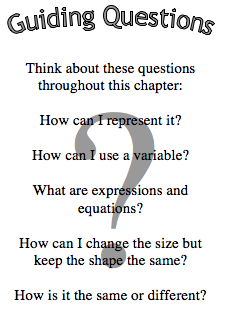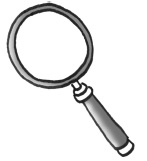# Variables and RatiosAre you ready to strengthen your pre-algebra skills?  One skill that is essential for algebra is figuring out unknown amounts.  In Section 4.1, you will begin to think about how to do so.  You will use variables to represent unknown quantities and will use what you know about a problem to find the value of these variables.

In Section 4.2, you will move from mystery numbers to a mystery mascot.  With your class, you will work to enlarge the mystery mascot.  Then you will learn how to enlarge or reduce figures while keeping their shapes the same.  You will use ratios to compare the side lengths of figures to determine if they are the same shape.

In this chapter, you will learn how to:

• Use variables to generalize and to represent unknown quantities.
• Write multiple expressions to describe a pattern and recognize whether the expressions are equivalent.
• Find the value of an algebraic expression when the value of the variable is known.
• Enlarge and reduce figures while maintaining their shapes.
• Use ratios to describe relationships between similar shapes.

## Mathematical Vocabulary• The following is a list of vocabulary found in this chapter.  Some of the words have been seen in a previous chapter.  The italicized words are new to this chapter.  Make sure that you are familiar with the terms below and know what they mean.  Go to the closure of the e textbook and click on the word for a “pop-up” definition. You might also add these words to your Toolkit so that you can reference them in the future.
•  algebraic expression enlarge equivalent expressions equivalent fractions equivalent ratios expression evaluate ratio reduce similar figures substitution variable vertex  (vertices)